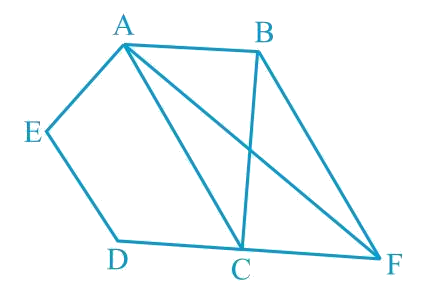In the verge of coronavirus pandemic, we are providing FREE access to our entire Online Curriculum to ensure Learning Doesn't STOP!

# Ex.9.3 Q11 Areas of Parallelograms and Triangles Solution - NCERT Maths Class 9

Go back to  'Ex.9.3'

## Question

In the given figure, $$ABCDE$$ is a pentagon. $$A$$ line through $$B$$ parallel to $$AC$$ meets $$DC$$ produced at $$F$$. Show that

(i) $$ar (ACB) = ar (ACF)$$

(ii) $$ar (AEDF) = ar (ABCDE)$$

Video Solution
Areas Of Parallelograms And Triangles
Ex 9.3 | Question 11

## Text Solution

What is known?

$$ABCDE$$ is a pentagon. $$A$$ line through $$B$$ parallel to $$AC$$ meets $$DC$$ produced at $$F.$$

What is unknown?

How we can show that

(i) $$ar (ACB) = ar (ACF)$$

(ii) $$ar (AEDF) = ar (ABCDE)$$

Reasoning:

We can use theorem for triangles $$ACB$$ and $$ACF$$, if two triangles are on same base and between same pair of parallel lines then both will have equal area. Now we can add area of quadrilateral $$ACDE$$ on both sides to get the second part required result.Steps:

(i) \begin{align}\rm \Delta ACB\, \rm and \,\Delta ACF\end{align} lie on the same base $$AC$$ and The same parallels $$AC$$ and $$BF$$.

According to Theorem 9.2: Two triangles on the same base (or equal bases) and between the same parallels are equal in area.

\begin{align}\text{Area}\; ( \Delta ACB ) = \text{Area}\; ( \Delta ACF )\end{align}

(ii) It can be observed that

\begin{align} {\text {ar}(\Delta {ACB})}\!=\!{\text {ar}(\Delta {ACF})} \end{align}

$\left[ \begin{array}{l}{{ar}}(\Delta {{ACB}}) + \\{{ar}}({{ACDE}})\end{array} \right] \!\!=\!\!\left[ \begin{array}{l}{{ar}}(\Delta {{ACF}}) + \\{{ar(ACDE) }}\end{array} \right]$

Adding Equal Areas  on both the sides.

\begin{align}\text{ar}\, (ABCDE)\!=\!\text{ar} \,(AEDF)\end{align}

Video Solution
Areas Of Parallelograms And Triangles
Ex 9.3 | Question 11